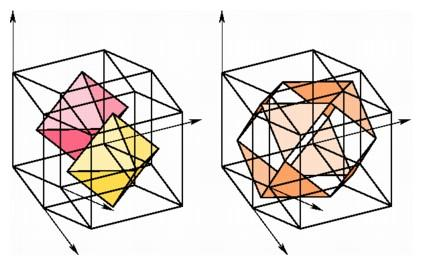Coordinates for Regular Polytopes

In the course of finding coordinates for the regular polyhedra, we have found coordinates for each of the three regular polytopes in n-dimensional space, namely the n-simplex, the n-cube, and the dual of the n-cube. For dimension n greater than four, these are the only possible regular figures, but in four-dimensional space there are three more regular polytopes, with 24, 120, or 600 cells. To prove that these polytopes actually exist, we can exhibit coordinates for their vertices.

The self-dual 24-cell, with octahedral faces, turns out to be the easiest to describe by coordinates, using a procedure similar to that for finding the dual. Instead of choosing points in the centers of highest-dimensional faces, we can choose points in the centers of edges or squares. This procedure always will yield a figure with a large amount of symmetry, but usually there will be cells of different shape. For example, in the three-dimensional cube, the 12 points found in the centers of edges are vertices of a cuboctahedron with 8 triangular faces and 6 square faces. The surprise is that the centers of the 24 squares in a hypercube turn out to be the vertices of a regular polytope in four-space, the self-dual 24-cell. If the coordinates of the vertices of a hypercube are all either 1 or -1, then a square in this hypercube is determined by the four vertices each having two coordinates fixed and the other two either 1 or -1. For example, one such square has the vertices (±1, ±1, 1, 1), and its midpoint will be (0, 0, 1, 1). The coordinates of the midpoints of all of the squares in the hypercube can be listed in six groups: (±1, ±1, 0, 0), (±1, 0, ±1, 0), (±1, 0, 0, ±1), (0, 0, ±1, ±1), (0, ±1, 0, ±1), and (0, ±1, ±1, 0). We obtain eight of the octahedra in the 24-cell by fixing one coordinate at 1 or -1, for example (±1, 0, 0, 1), (0, ±1, 0, 1), and (0, 0, ±1, 1). The other 16 octahedra are obtained by choosing a vertex of the hypercube and replacing two of its coordinates by 0 in six different ways; for example, (1, -1, -1, 1) yields the vertices (1, -1, 0, 0), (1, 0, -1, 0), (1, 0, 0, 1), (0, 0, -1, 1), (0, -1, 0, 1), and (0, -1, -1, 0).Slices of the centered 24-cell with final coordinate 1 or -1 are octahedra (left), while the slice with final coordinate 0 contains 12 vertices forming the vertices of a cuboctahedron (right).

By carefully exploiting their symmetry, it is possible to choose coordinates for all 600 vertices of the 12-cell and for all 120 vertices of the 600-cell. We can instruct a graphics computer to display the image of any of these polytopes in the plane, or we can use the animation capabilities of the machine to create a motion picture of the image in three-space. There is a real art to choosing coordinates in a way that transmits the maximum amount of visual information. Some of the mathematical principles underlying this art are contained in the several books of the Canadian geometer H. S. M. Coxeter, one of the foremost developers of the theory of polyhedra and polytopes. He wrote is first paper in the subject in 1923 at the age of 16.